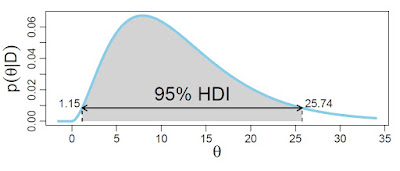## Friday, February 23, 2018

### Run MCMC to achieve effective sample size (ESS) of 10,000

There's a great new book by Farrell and Lewandowsky, Computational Modeling of Cognition and Behavior (at the publisher, at Amazon.com), that includes some chapters on Bayesian methods. Each chapter includes a little "in vivo" commentary by an outside contributor. My commentary accompanies their chapter regarding JAGS. The commentary is posted here in a succession of three blog posts; this is 1 of 3. Do check out their book!

Run MCMC to achieve effective sample size (ESS) of 10,000

Bayesian analysis of complex models is possible only by virtue of modern software that takes an abstract model specification and returns a representation of the posterior distribution. In software that uses Markov-chain Monte Carlo (MCMC) methods, such as JAGS, the representation is inherently noisy. The random noise from MCMC tends to cancel out as the chain gets longer and longer. But different aspects of the posterior distribution are differently affected by noise. A relatively stable aspect is the median value of the chain. The median tends to stabilize relatively quickly, that is, with relatively shorter chains, because the median is usually in a high-density region of the posterior and the value of the median does not depend on the distance to outliers (unlike the mean). But other crucial aspects of the posterior distribution tend to need longer chains to achieve stable values.

In particular, a crucial aspect of a parameter distribution is its width. Narrower distributions connote more certainty in the estimate of the parameter. A very useful indicator of the width of a distribution is its 95% highest density interval (HDI). Parameter values within the 95% HDI have higher probability density than parameter values outside the HDI, and the parameter values inside the 95% HDI have a total probability of 95%. An example of an HDI is illustrated in Figure 1.Figure 1. Example of a 95% highest density interval (HDI). On the axes of the graph, θ denotes a parameter in the model, and p(θ|D) denotes the posterior distribution of that parameter. The limits of the HDI are marked by the ends of the double-headed arrow. Any value of θ within the HDI has higher probability density than any value outside the HDI. The mass within the 95% HDI, shaded by gray in the figure, is 95%.
Because the limits of an HDI are usually in the low-density tails of the distribution, there are relatively few steps in the MCMC chain near the limits. Therefore it takes a long chain to generate sufficiently many representative values of the parameter to stabilize the estimate of the HDI limits.

How long of a chain is needed to produce stable estimates of the 95% HDI? One useful heuristic answer is 10,000 independent steps. The rationale for the heuristic is explained in Section 7.5.2 of Kruschke (2015). Note that the requirement is 10,000 independent steps. Unfortunately, most MCMC chains are strongly autocorrelated, meaning that successive steps are near each other, and are not independent. Therefore we need a measure of chain length that takes into account the autocorrelation of the chain. Such a measure is called the effective sample size (ESS), for which a formal definition is provided in Section 7.5.2 of Kruschke (2015).

ESS is computed in R by the effectiveSize function (which is in the coda package, which in turn is part of the rjags package for JAGS). For example, suppose we have generated an MCMC chain using the rjags function, coda.samples, and the resulting object is called mcmcfin. Then we can find the ESS of the parameters by typing effectiveSize(mcmcfin).

It is crucial to realize that (i) the ESS will usually be much less than the number of steps in the MCMC chain, and (ii) every parameter in a multi-parameter model has a different ESS. Some parameters might have large ESS while others have small ESS. Moreover, combinations of parameters, such as a difference of two means, can have quite different ESS than the separate parameters. Therefore it is important to check the ESS of every parameter of interest, and the ESS of any interesting parameter combinations.

Stay tuned for Parts 2 and 3...

1.1.